# OECD Non-Financial Corporations Debt to Surplus Ratio Analysis 2 - making various type plots with ggplot() + geom_~~~ using R.UnsplashJ Cruikshankが撮影した写真

This post is following of the above post.

In the previous post, I load CSV file data into R. Then, let's make some basic graphs using ggplot2 package.

Scatter plot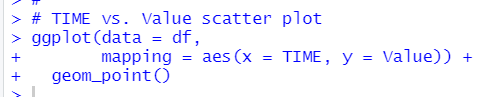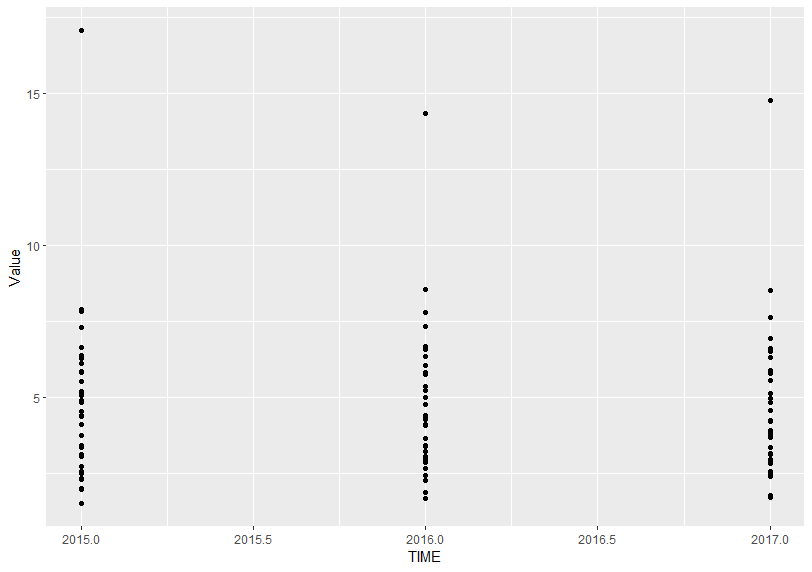ggplot() + geom_point() makes scatter plot.

Boxplot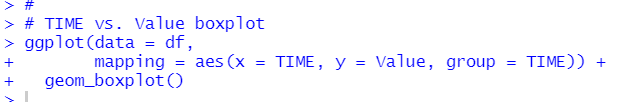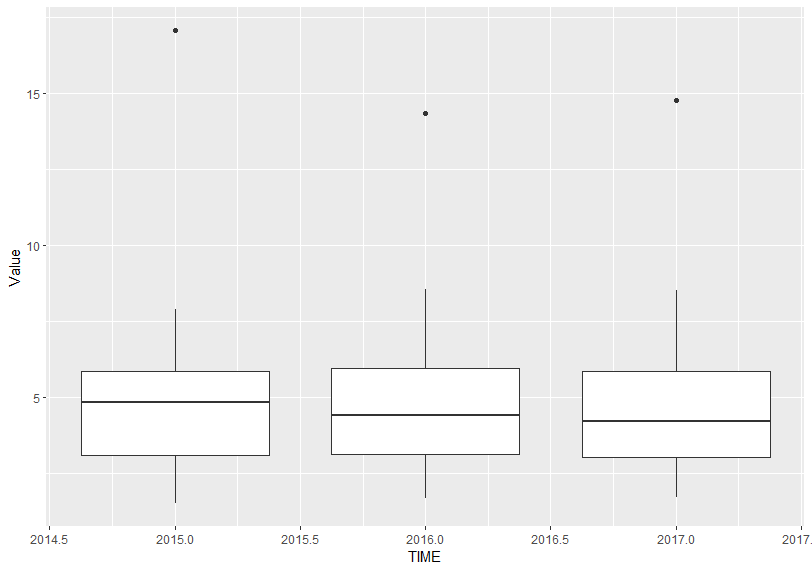ggplot() + geom_boxplot() makes boxplot

Line plot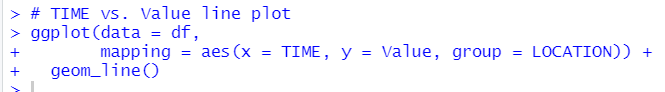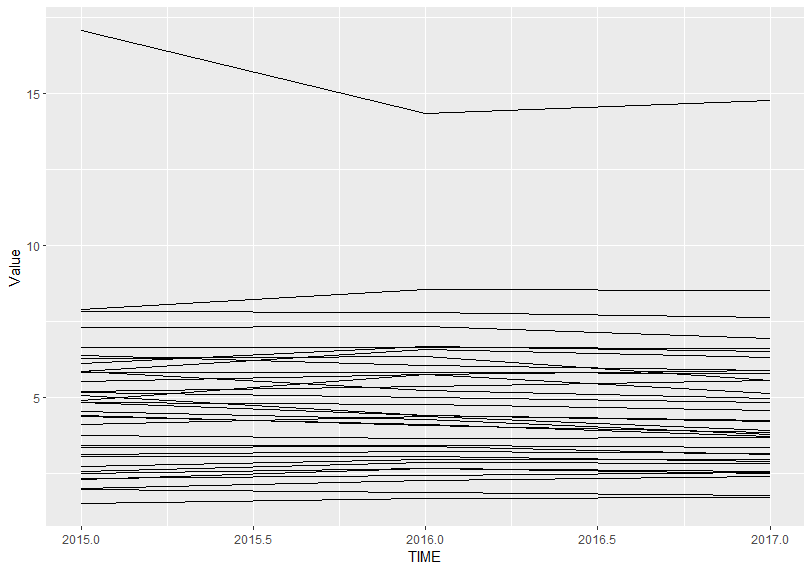ggplot() + geom_line() makes line plot.

Histogram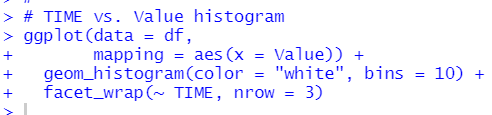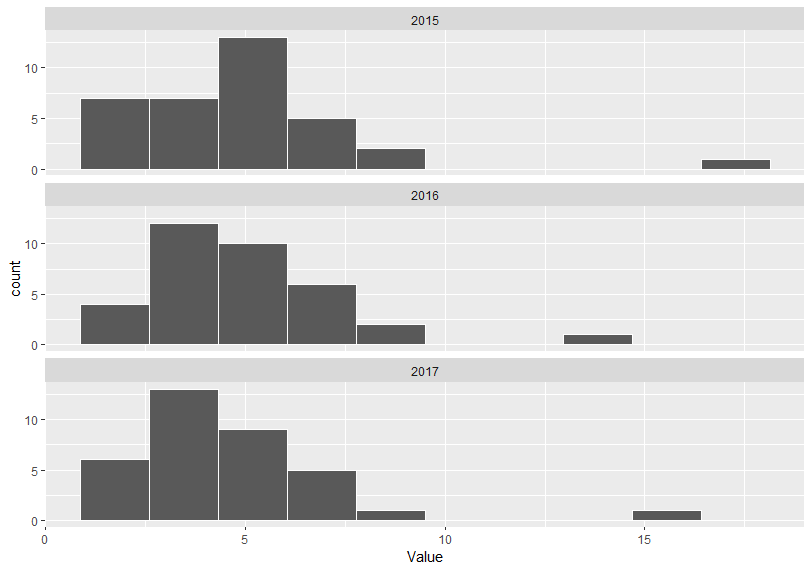ggplot() + geom_histogram() makes a histogram.

I use color = "white" to make border lines whilte and bins = 10 to make number of bins 10. Then, I use facet_wrap() function to make histogram for each TIME.

Violin plot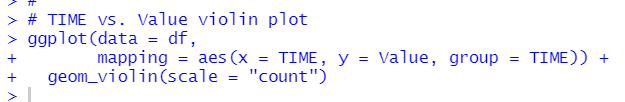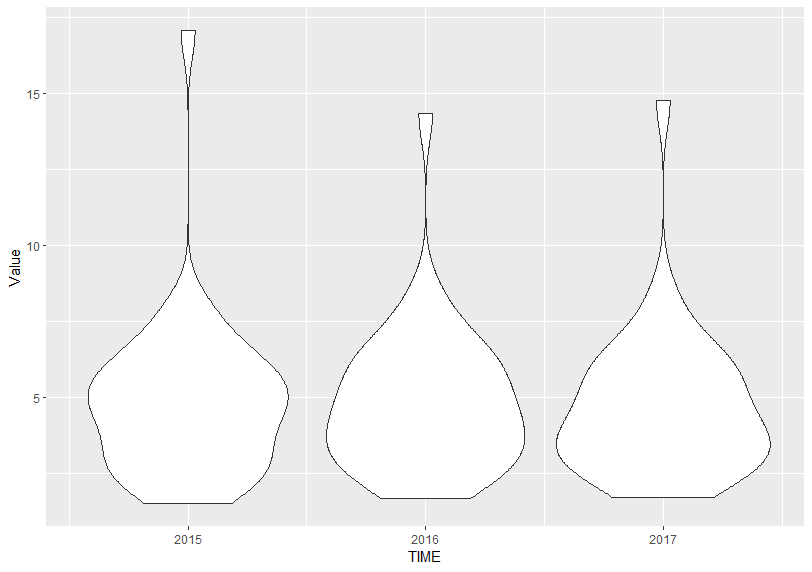ggplot() + geom_violin() makes violin plot.

Density plot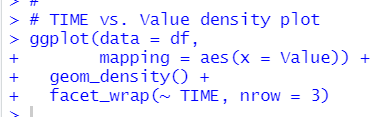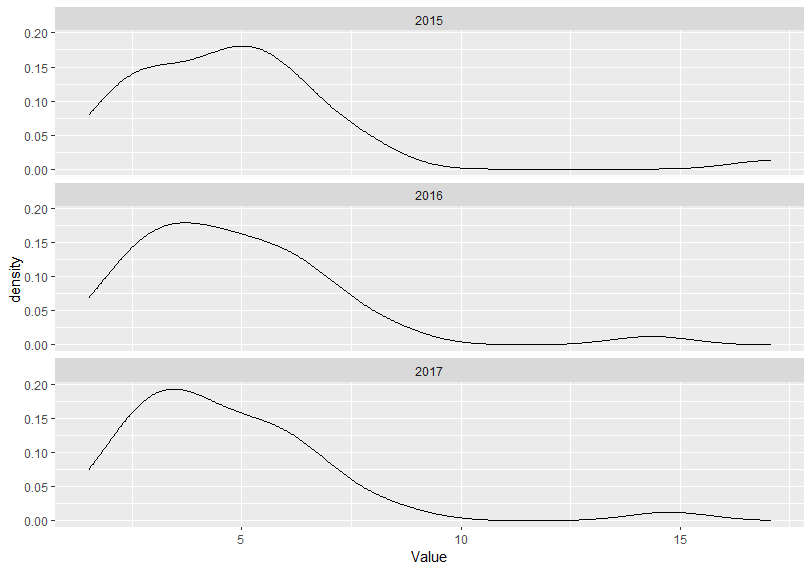ggplot() + geom_density() makes density plots. It is like histogram.

According to above plots, I find Debt to Surplus Ratio has similar distribution pattern for 2015, 2016 and 2017, almost LOCATION has less than 10 value.

That's it. Thank you!

The next post is

To read from the 1st post,